# Class B amplifier has automatic bias

## Texas Instruments TLV271

Pierre Corbeil

EDN

Class B amplifiers are prone to crossover distortion, which occurs in the output stage in which conduction transfers from one transistor to the other. To prevent crossover distortion, a bias current must flow in both transistors simultaneously. The bias current prevents both transistors from turning off in the transition region. Classic bias circuits keep a constant dc polarization voltage between the bases of the two transistors. Often manually adjusted, it keeps the two transistors on the edge of conduction when there is no signal present. Such a circuit is sensitive to temperature and needs some form of compensation to prevent thermal runaway, which can lead to failure. Figure 1 shows an approach in which automatic bias eliminates the problem.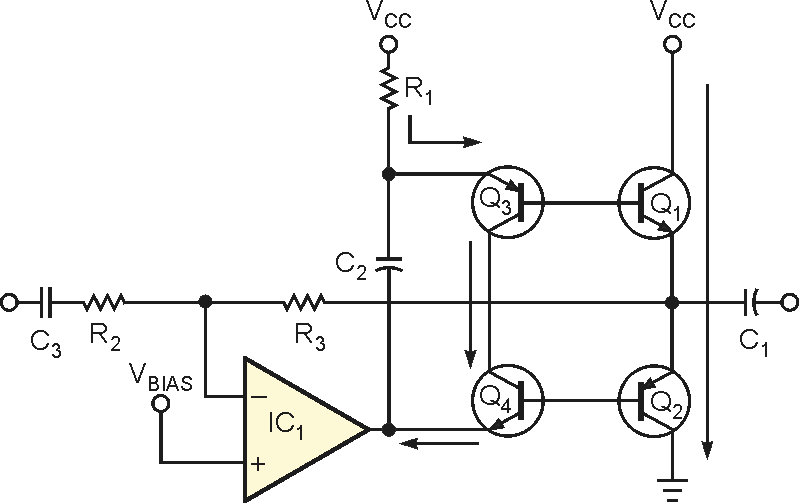Figure 1. A bias current flows in the transistors that prevents Q1 and Q2 from being off simultaneously.

In this Class B amplifier, R1 sets the bias current at idle mode with no signal. Emitter current IE for Q3 is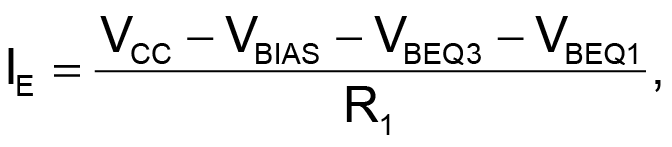where

VCC is the power-supply voltage,
VBIAS is the dc voltage on the emitters of Q1 and Q2,
VBEQ3 is the base-to-emitter voltage of Q3,
VBEQ1 is the base-to-emitter voltage of Q1.

Q1 and Q2 mirror this current because Q1 and Q3 share the same base current, as do Q2 and Q4. Assuming that the four transistors are perfectly matched, all of them have the same base current and the same collector current, so the emitters of Q1 and Q2 precisely mirror the current in R1. Transistor matching is unnecessary, however. With unmatched transistors, either Q3 or Q4 must operate in saturation, and, because the mirror effect depends on the transistors’ current gain, hFE, the difference between Q1/Q2 bias current and the current in R1 can be significant. This circuit automatically adjusts the voltage on C2 to compensate for temperature and the transistors’ characteristics.

When a signal is present, the current gain is the hFE of output transistor Q1 or Q2 (the same as for a classic Class B amplifier). On the positive part of the signal, Q1 carries the load current. Because the base current increases, Q3 enters saturation. On the negative part of the signal, Q2 carries the load current and Q4 saturates.

 а)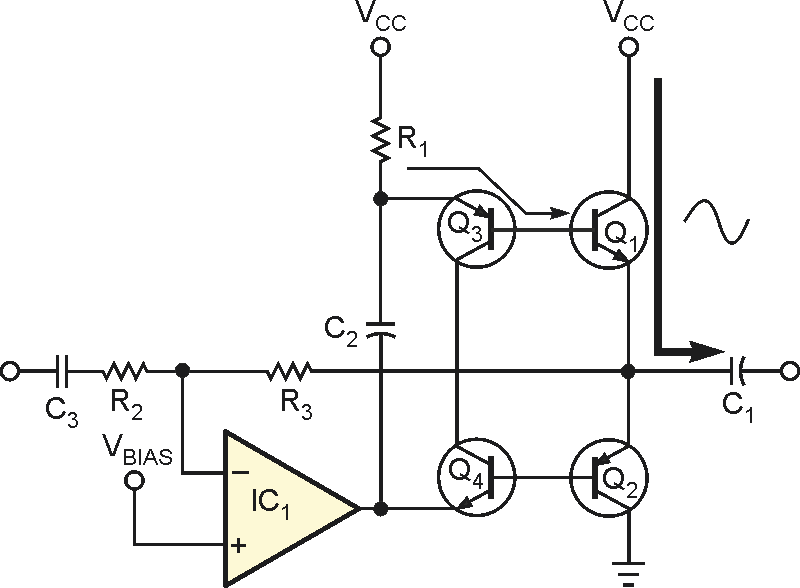b)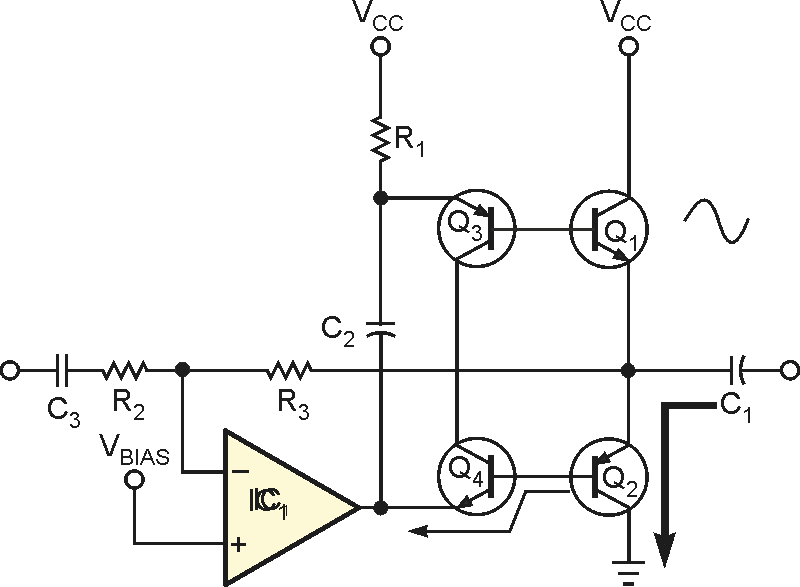Figure 2. On a positive half-cycle, current flows from Q1 through C1 to a
load (a). On a negative half-cycle, current flows through Q2 (b).

Figure 2 shows the ac-current path. The maximum average load current IL is the idle current in R1 times the current gain of Q1 times two. The op amp must be able to sink the base current IB of Q2: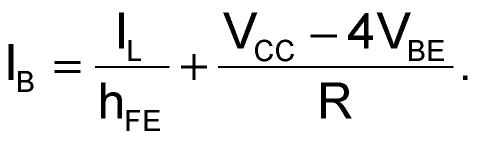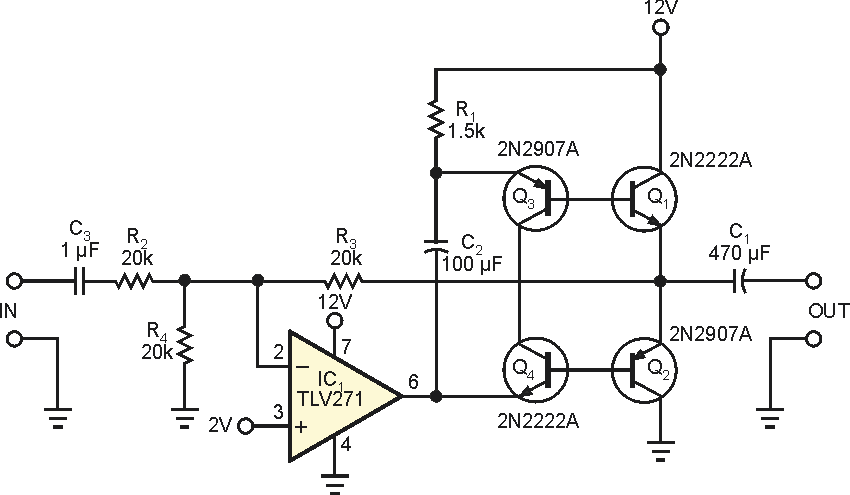Figure 3. A typical application of this Class B circuit is a headphone amplifier.

A typical application of this Class B amplifier delivers 0.25 W into 8 Ω (Figure 3). Figure 4 shows the total harmonic distortion over the 45-Hz to 50-kHz band – that is, 1 V rms into 8 Ω.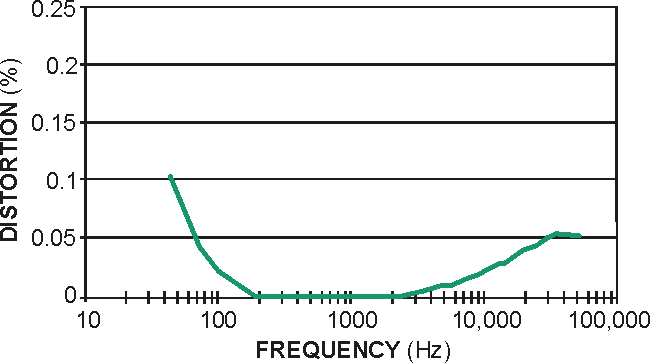Figure 4. This graph shows distortion as measured on the circuit of Figure 3.

EDN